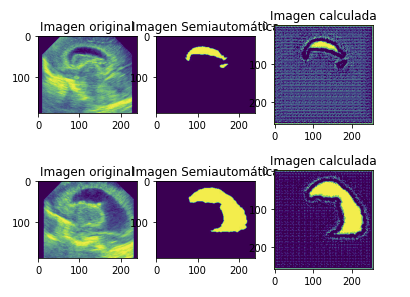# UNET strange segmentation

I’m trying to build an UNET implementation to segment some cerebral images.

This is the implementation of the net:

``````class ConvBlock(nn.Module):
def __init__(self,input,output):
super(ConvBlock,self).__init__()
self.conv = nn.Sequential(
nn.BatchNorm2d(output),
nn.ReLU(inplace=True),
nn.BatchNorm2d(output),
nn.ReLU(inplace=True)
)

def forward(self,x):
x = self.conv(x)
return x

class Unet (nn.Module):
def __init__(self,numberClasses = 2,dropout = 0.5, lastLayer = "SIGMOID"):
super(Unet, self).__init__()

self.CB1 = ConvBlock(1,64)
self.CB2 = ConvBlock(64,128)
self.CB3 = ConvBlock(128,256)
self.CB4 = ConvBlock(256,512)

self.CB5 = ConvBlock(512,1024)

self.CB6 = ConvBlock(1024,512)
self.CB7 = ConvBlock(512,256)
self.CB8 = ConvBlock(256,128)
self.CB9 = ConvBlock(128,64)

self.pool = nn.MaxPool2d(2)

self.dropout = nn.Dropout(dropout)

self.UC1 = nn.ConvTranspose2d(1024,512,2,stride = 2)
self.UC2 = nn.ConvTranspose2d(512,256,2,stride = 2)
self.UC3 = nn.ConvTranspose2d(256,128,2,stride = 2)
self.UC4 = nn.ConvTranspose2d(128,64,2,stride = 2)

self.FC = nn.Conv2d(64,numberClasses,1)

if lastLayer == "SIGMOID":
self.lastLayer = nn.Sigmoid()
elif lastLayer == "SOFTMAX":
self.lastLayer = nn.Softmax(dim = 1)

def forward(self,x):
# DOWN SAMPLING
C1 = self.CB1(x)
x = C1.clone()
x = self.pool(x)
x = self.dropout(x)

C2 = self.CB2(x)
x = C2.clone()
x = self.pool(x)
x = self.dropout(x)

C3 = self.CB3(x)
x = C3.clone()
x = self.pool(x)
x = self.dropout(x)

C4 = self.CB4(x)
x = C4.clone()
x = self.pool(x)
x = self.dropout(x)

x = self.CB5(x)

# UP SAMPLING
x = self.UC1(x)

x = torch.cat((x,C4),dim=1)
x = self.CB6(x)
x = self.dropout(x)

x = self.UC2(x)
x = torch.cat((x,C3),dim=1)
x = self.CB7(x)
x = self.dropout(x)

x = self.UC3(x)
x = torch.cat((x,C2),dim=1)
x = self.CB8(x)
x = self.dropout(x)

x = self.UC4(x)
x = torch.cat((x,C1),dim=1)
x = self.CB9(x)

x = self.FC(x)

x = self.lastLayer(x)

return x
``````

I’m using a BCE loss with weighted classes, where class 0 is multiplied by 0.2 and 1 by 0.8. This is the best configuration I have found, but it still doesn’t work.

Here are some examples, comparing ground truth, expected segmentation and final results calculated by the net:The shape seems to be correct, but I need better results, because I will count every single pixel later. If I keep training the model I get an empty output. What should I do? Apply some kind of morphological transformation?